# paretosearch

Find points in Pareto set

Since R2018b

## Syntax

``x = paretosearch(fun,nvars)``
``x = paretosearch(fun,nvars,A,b)``
``x = paretosearch(fun,nvars,A,b,Aeq,beq)``
``x = paretosearch(fun,nvars,A,b,Aeq,beq,lb,ub)``
``x = paretosearch(fun,nvars,A,b,Aeq,beq,lb,ub,nonlcon)``
``x = paretosearch(fun,nvars,A,b,Aeq,beq,lb,ub,nonlcon,options)``
``x = paretosearch(problem)``
``````[x,fval] = paretosearch(___)``````
``````[x,fval,exitflag,output] = paretosearch(___)``````
``````[x,fval,exitflag,output,residuals] = paretosearch(___)``````

## Description

example

````x = paretosearch(fun,nvars)` finds nondominated points of the multiobjective function `fun`. The `nvars` argument is the dimension of the optimization problem (number of decision variables).```

example

````x = paretosearch(fun,nvars,A,b)` finds nondominated points subject to the linear inequalities `A*x` ≤ `b`. See Linear Inequality Constraints.```
````x = paretosearch(fun,nvars,A,b,Aeq,beq)` finds nondominated points subject to the linear constraints `Aeq*x` = `beq` and `A*x` ≤ `b`. If no linear inequalities exist, set `A = []` and ```b = []```.```

example

````x = paretosearch(fun,nvars,A,b,Aeq,beq,lb,ub)` defines a set of lower and upper bounds on the design variables in `x`, so that `x` is always in the range `lb `≤` x `≤` ub`. If no linear equalities exist, set `Aeq = []` and ```beq = []```. If `x(i)` has no lower bound, set ```lb(i) = -Inf```. If `x(i)` has no upper bound, set `ub(i) = Inf`.```

example

````x = paretosearch(fun,nvars,A,b,Aeq,beq,lb,ub,nonlcon)` applies the nonlinear inequalities `c(x)` defined in `nonlcon`. The `paretosearch` function finds nondominated points such that `c(x) ≤ 0`. If no bounds exist, set `lb = []`, `ub = []`, or both. NoteCurrently, `paretosearch` does not support nonlinear equality constraints `ceq(x) = 0`. ```

example

````x = paretosearch(fun,nvars,A,b,Aeq,beq,lb,ub,nonlcon,options)` finds nondominated points with the optimization options specified in `options`. Use `optimoptions` to set these options. If there are no nonlinear inequality or equality constraints, set `nonlcon = []`.```
````x = paretosearch(problem)` finds the nondominated points for `problem`, where `problem` is a structure described in `problem`.```

example

``````[x,fval] = paretosearch(___)```, for any input variables, returns the matrix `fval`, the value of all the objective functions in `fun` for all the solutions (rows) in `x`. The output `fval` has `nf` columns, where `nf` is the number of objectives, and has the same number of rows as `x`.```

example

``````[x,fval,exitflag,output] = paretosearch(___)``` also returns `exitflag`, an integer identifying the reason the algorithm stopped, and `output`, a structure that contains information about the solution process.```

example

``````[x,fval,exitflag,output,residuals] = paretosearch(___)``` also returns `residuals`, a structure containing the constraint values at the solution points `x`.```

## Examples

collapse all

Find points on the Pareto front of a two-objective function of a two-dimensional variable.

```fun = @(x)[norm(x-[1,2])^2;norm(x+[2,1])^2]; rng default % For reproducibility x = paretosearch(fun,2);```
```Pareto set found that satisfies the constraints. Optimization completed because the relative change in the volume of the Pareto set is less than 'options.ParetoSetChangeTolerance' and constraints are satisfied to within 'options.ConstraintTolerance'. ```

Plot the solution as a scatter plot.

```plot(x(:,1),x(:,2),'m*') xlabel('x(1)') ylabel('x(2)')```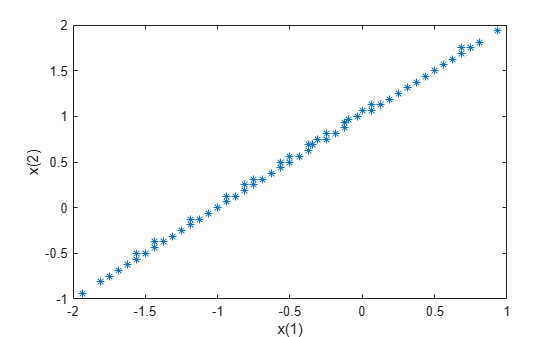Theoretically, the solution of this problem is a straight line from `[-2,-1]` to `[1,2]`. `paretosearch` returns evenly-spaced points close to this line.

Create a Pareto front for a two-objective problem in two dimensions subject to the linear constraint `x(1) + x(2) <= 1`.

```fun = @(x)[norm(x-[1,2])^2;norm(x+[2,1])^2]; A = [1,1]; b = 1; rng default % For reproducibility x = paretosearch(fun,2,A,b);```
```Pareto set found that satisfies the constraints. Optimization completed because the relative change in the volume of the Pareto set is less than 'options.ParetoSetChangeTolerance' and constraints are satisfied to within 'options.ConstraintTolerance'. ```

Plot the solution as a scatter plot.

```plot(x(:,1),x(:,2),'m*') xlabel('x(1)') ylabel('x(2)')```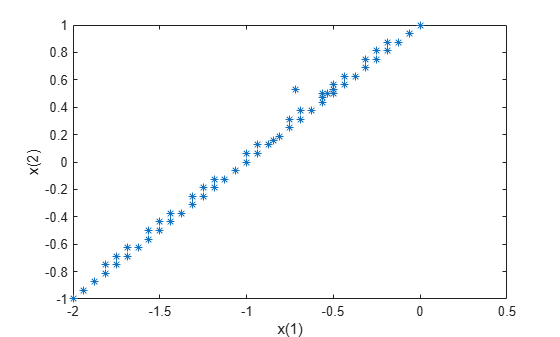Theoretically, the solution of this problem is a straight line from `[-2,-1]` to `[0,1]`. `paretosearch` returns evenly-spaced points close to this line.

Create a Pareto front for a two-objective problem in two dimensions subject to the bounds `x(1) >= 0` and `x(2) <= 1`.

```fun = @(x)[norm(x-[1,2])^2;norm(x+[2,1])^2]; lb = [0,-Inf]; % x(1) >= 0 ub = [Inf,1]; % x(2) <= 1 rng default % For reproducibility x = paretosearch(fun,2,[],[],[],[],lb,ub);```
```Pareto set found that satisfies the constraints. Optimization completed because the relative change in the volume of the Pareto set is less than 'options.ParetoSetChangeTolerance' and constraints are satisfied to within 'options.ConstraintTolerance'. ```

Plot the solution as a scatter plot.

```plot(x(:,1),x(:,2),'m*') xlabel('x(1)') ylabel('x(2)')```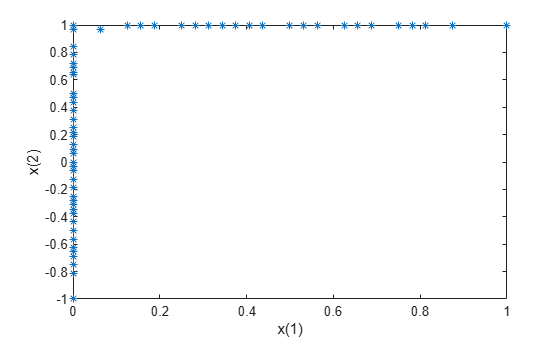All of the solution points are on the constraint boundaries `x(1) = 0` or `x(2) = 1`.

Create a Pareto front for a two-objective problem in two dimensions subject to bounds `-1.1 <= x(i) <= 1.1` and the nonlinear constraint `norm(x)^2 <= 1.2`. The nonlinear constraint function appears at the end of this example, and works if you run this example as a live script. To run this example otherwise, include the nonlinear constraint function as a file on your MATLAB® path.

To better see the effect of the nonlinear constraint, set options to use a large Pareto set size.

```rng default % For reproducibility fun = @(x)[norm(x-[1,2])^2;norm(x+[2,1])^2]; lb = [-1.1,-1.1]; ub = [1.1,1.1]; options = optimoptions('paretosearch','ParetoSetSize',200); x = paretosearch(fun,2,[],[],[],[],lb,ub,@circlecons,options);```
```Pareto set found that satisfies the constraints. Optimization completed because the relative change in the volume of the Pareto set is less than 'options.ParetoSetChangeTolerance' and constraints are satisfied to within 'options.ConstraintTolerance'. ```

Plot the solution as a scatter plot. Include a plot of the circular constraint boundary.

```figure plot(x(:,1),x(:,2),'k*') xlabel('x(1)') ylabel('x(2)') hold on rectangle('Position',[-1.2 -1.2 2.4 2.4],'Curvature',1,'EdgeColor','r') xlim([-1.2,0.5]) ylim([-0.5,1.2]) axis square hold off```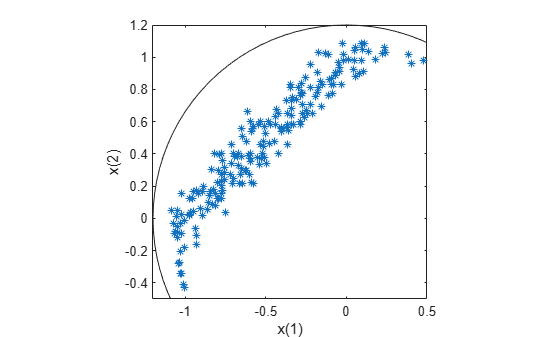The solution points that have positive `x(1)` values or negative `x(2)` values are close to the nonlinear constraint boundary.

```function [c,ceq] = circlecons(x) ceq = []; c = norm(x)^2 - 1.2; end```

To monitor the progress of `paretosearch`, specify the `'psplotparetof'` plot function.

```fun = @(x)[norm(x-[1,2])^2;norm(x+[2,1])^2]; options = optimoptions('paretosearch','PlotFcn','psplotparetof'); lb = [-4,-4]; ub = -lb; x = paretosearch(fun,2,[],[],[],[],lb,ub,[],options);```
```Pareto set found that satisfies the constraints. Optimization completed because the relative change in the volume of the Pareto set is less than 'options.ParetoSetChangeTolerance' and constraints are satisfied to within 'options.ConstraintTolerance'. ```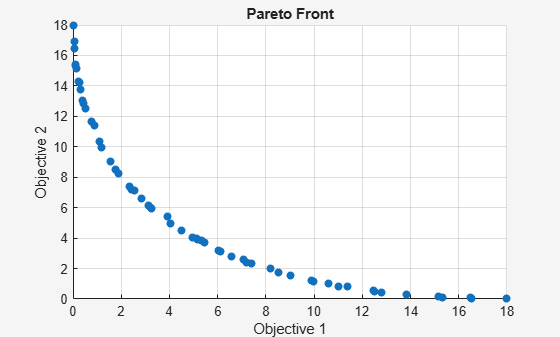The solution looks like a quarter-circular arc with radius 18, which can be shown to be the analytical solution.

Obtain the Pareto front in both function space and parameter space by calling `paretosearch` with both the `x` and `fval` outputs. Set options to plot the Pareto set in both function space and parameter space.

```fun = @(x)[norm(x-[1,2])^2;norm(x+[2,1])^2]; lb = [-4,-4]; ub = -lb; options = optimoptions('paretosearch','PlotFcn',{'psplotparetof' 'psplotparetox'}); rng default % For reproducibility [x,fval] = paretosearch(fun,2,[],[],[],[],lb,ub,[],options);```
```Pareto set found that satisfies the constraints. Optimization completed because the relative change in the volume of the Pareto set is less than 'options.ParetoSetChangeTolerance' and constraints are satisfied to within 'options.ConstraintTolerance'. ```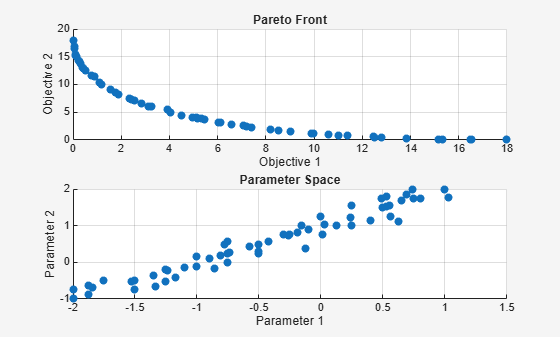The analytical solution in objective function space is a quarter-circular arc of radius 18. In parameter space, the analytical solution is a straight line from `[-2,-1]` to `[1,2]`. The solution points are close to the analytical curves.

Set options to monitor the Pareto set solution process. Also, obtain more outputs from `paretosearch` to enable you to understand the solution process.

```options = optimoptions('paretosearch','Display','iter',... 'PlotFcn',{'psplotparetof' 'psplotparetox'}); fun = @(x)[norm(x-[1,2])^2;norm(x+[2,1])^2]; lb = [-4,-4]; ub = -lb; rng default % For reproducibility [x,fval,exitflag,output] = paretosearch(fun,2,[],[],[],[],lb,ub,[],options);```
```Iter F-count NumSolutions Spread Volume 0 60 11 - 3.7872e+02 1 386 12 7.6126e-01 3.4654e+02 2 702 27 9.5232e-01 2.9452e+02 3 1029 27 6.6332e-02 2.9904e+02 4 1357 36 1.3874e-01 3.0070e+02 5 1690 37 1.5379e-01 3.0200e+02 6 2014 50 1.7828e-01 3.0252e+02 7 2214 59 1.8536e-01 3.0320e+02 8 2344 60 1.9435e-01 3.0361e+02 9 2464 60 2.1055e-01 3.0388e+02 Pareto set found that satisfies the constraints. Optimization completed because the relative change in the volume of the Pareto set is less than 'options.ParetoSetChangeTolerance' and constraints are satisfied to within 'options.ConstraintTolerance'. ````fprintf('Exit flag %d.\n',exitflag)`
```Exit flag 1. ```
`disp(output)`
``` iterations: 10 funccount: 2464 volume: 303.6076 averagedistance: 0.0250 spread: 0.2105 maxconstraint: 0 message: 'Pareto set found that satisfies the constraints. ...' rngstate: [1x1 struct] ```

Obtain and examine the Pareto front constraint residuals. Create a problem with the linear inequality constraint `sum(x) <= -1/2` and the nonlinear inequality constraint `norm(x)^2 <= 1.2`. For improved accuracy, use 200 points on the Pareto front, and a `ParetoSetChangeTolerance` of `1e-7`, and give the natural bounds `-1.2 <= x(i) <= 1.2`.

The nonlinear constraint function appears at the end of this example, and works if you run this example as a live script. To run this example otherwise, include the nonlinear constraint function as a file on your MATLAB® path.

```fun = @(x)[norm(x-[1,2])^2;norm(x+[2,1])^2]; A = [1,1]; b = -1/2; lb = [-1.2,-1.2]; ub = -lb; nonlcon = @circlecons; rng default % For reproducibility options = optimoptions('paretosearch','ParetoSetChangeTolerance',1e-7,... 'PlotFcn',{'psplotparetof' 'psplotparetox'},'ParetoSetSize',200);```

Call `paretosearch` using all outputs.

`[x,fval,exitflag,output,residuals] = paretosearch(fun,2,A,b,[],[],lb,ub,nonlcon,options);`
```Pareto set found that satisfies the constraints. Optimization completed because the relative change in the volume of the Pareto set is less than 'options.ParetoSetChangeTolerance' and constraints are satisfied to within 'options.ConstraintTolerance'. ```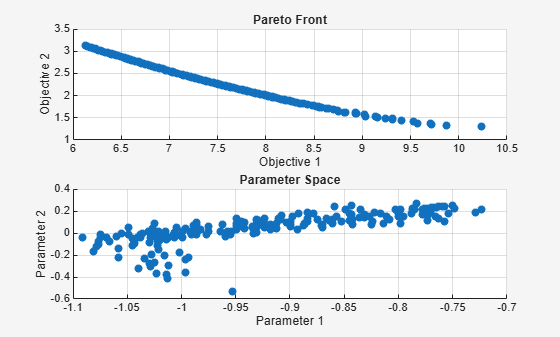The inequality constraints reduce the size of the Pareto set compared to an unconstrained set. Examine the returned residuals.

`fprintf('The maximum linear inequality constraint residual is %f.\n',max(residuals.ineqlin))`
```The maximum linear inequality constraint residual is 0.000000. ```
`fprintf('The maximum nonlinear inequality constraint residual is %f.\n',max(residuals.ineqnonlin))`
```The maximum nonlinear inequality constraint residual is -0.002142. ```

The maximum returned residuals are negative, meaning that all the returned points are feasible. The maximum returned residuals are close to zero, meaning that each constraint is active for some points.

```function [c,ceq] = circlecons(x) ceq = []; c = norm(x)^2 - 1.2; end```

## Input Arguments

collapse all

Objective functions to optimize, specified as a function handle or function name.

`fun` is a function that accepts a real row vector of doubles `x` of length `nvars` and returns a real vector `F(x)` of objective function values. For details on writing `fun`, see Compute Objective Functions.

If you set the `UseVectorized` option to `true`, then `fun` accepts a matrix of size `n`-by-`nvars`, where the matrix represents `n` individuals. `fun` returns a matrix of size `n`-by-`m`, where `m` is the number of objective functions. See Vectorize the Fitness Function.

Example: `@(x)[sin(x),cos(x)]`

Data Types: `char` | `function_handle` | `string`

Number of variables, specified as a positive integer. The solver passes row vectors of length `nvars` to `fun`.

Example: `4`

Data Types: `double`

Linear inequality constraints, specified as a real matrix. `A` is an `M`-by-`nvars` matrix, where `M` is the number of inequalities.

`A` encodes the `M` linear inequalities

`A*x <= b`,

where `x` is the column vector of `nvars` variables `x(:)`, and `b` is a column vector with `M` elements.

For example, to specify

x1 + 2x2 ≤ 10
3x1 + 4x2 ≤ 20
5x1 + 6x2 ≤ 30,

give these constraints:

```A = [1,2;3,4;5,6]; b = [10;20;30];```

Example: To specify that the control variables sum to 1 or less, give the constraints `A = ones(1,N)` and `b = 1`.

Data Types: `double`

Linear inequality constraints, specified as a real vector. `b` is an `M`-element vector related to the `A` matrix. If you pass `b` as a row vector, solvers internally convert `b` to the column vector `b(:)`.

`b` encodes the `M` linear inequalities

`A*x <= b`,

where `x` is the column vector of `N` variables `x(:)`, and `A` is a matrix of size `M`-by-`N`.

For example, to specify

x1 + 2x2 ≤ 10
3x1 + 4x2 ≤ 20
5x1 + 6x2 ≤ 30,

give these constraints:

```A = [1,2;3,4;5,6]; b = [10;20;30];```

Example: To specify that the control variables sum to 1 or less, give the constraints `A = ones(1,N)` and `b = 1`.

Data Types: `double`

Linear equality constraints, specified as a real matrix. `Aeq` is an `Me`-by-`nvars` matrix, where `Me` is the number of equalities.

`Aeq` encodes the `Me` linear equalities

`Aeq*x = beq`,

where `x` is the column vector of `N` variables `x(:)`, and `beq` is a column vector with `Me` elements.

For example, to specify

x1 + 2x2 + 3x3 = 10
2x1 + 4x2 + x3 = 20,

give these constraints:

```Aeq = [1,2,3;2,4,1]; beq = [10;20];```

Example: To specify that the control variables sum to 1, give the constraints ```Aeq = ones(1,N)``` and `beq = 1`.

Data Types: `double`

Linear equality constraints, specified as a real vector. `beq` is an `Me`-element vector related to the `Aeq` matrix. If you pass `beq` as a row vector, solvers internally convert `beq` to the column vector `beq(:)`.

`beq` encodes the `Me` linear equalities

`Aeq*x = beq`,

where `x` is the column vector of `N` variables `x(:)`, and `Aeq` is a matrix of size `Meq`-by-`N`.

For example, to specify

x1 + 2x2 + 3x3 = 10
2x1 + 4x2 + x3 = 20,

give these constraints:

```Aeq = [1,2,3;2,4,1]; beq = [10;20];```

Example: To specify that the control variables sum to 1, give the constraints ```Aeq = ones(1,N)``` and `beq = 1`.

Data Types: `double`

Lower bounds, specified as a real vector or array of doubles. `lb` represents the lower bounds element-wise in `lb `` x `` ub`.

Internally, `paretosearch` converts an array `lb` to the vector `lb(:)`.

Example: `lb = [0;-Inf;4]` means `x(1) ≥ 0`, `x(3) ≥ 4`.

Data Types: `double`

Upper bounds, specified as a real vector or array of doubles. `ub` represents the upper bounds element-wise in `lb `` x `` ub`.

Internally, `paretosearch` converts an array `ub` to the vector `ub(:)`.

Example: `ub = [Inf;4;10]` means `x(2) ≤ 4`, `x(3) ≤ 10`.

Data Types: `double`

Nonlinear constraints, specified as a function handle or function name. `nonlcon` is a function that accepts a row vector `x` and returns two row vectors, `c(x)` and `ceq(x)`.

• `c(x)` is the row vector of nonlinear inequality constraints at `x`. The `paretosearch` function attempts to satisfy `c(x) <= 0` for all entries of `c`.

• `ceq(x)` must return `[]`, because currently `paretosearch` does not support nonlinear equality constraints.

If you set the `UseVectorized` option to `true`, then `nonlcon` accepts a matrix of size `n`-by-`nvars`, where the matrix represents `n` individuals. `nonlcon` returns a matrix of size `n`-by-`mc` in the first argument, where `mc` is the number of nonlinear inequality constraints. See Vectorize the Fitness Function.

For example, `x = paretosearch(@myfun,nvars,A,b,Aeq,beq,lb,ub,@mycon)`, where `mycon` is a MATLAB® function such as the following:

```function [c,ceq] = mycon(x) c = ... % Compute nonlinear inequalities at x. ceq = [] % No nonlinear equalities at x.```

Data Types: `char` | `function_handle` | `string`

Optimization options, specified as the output of `optimoptions` or as a structure.

`{}` denotes the default value. See option details in Pattern Search Options.

Options for `paretosearch`

OptionDescriptionValues

`ConstraintTolerance`

Tolerance on constraints.

For an options structure, use `TolCon`.

Positive scalar | `{1e-6}`

`Display`

Level of display.

`'off'` | `'iter'` | `'diagnose'` | `{'final'}`

`InitialPoints`

Initial points for `paretosearch`. Use one of these data types:

• Matrix with `nvars` columns, where each row represents one initial point.

• Structure containing the following fields (all fields are optional except `X0`):

• `X0` — Matrix with `nvars` columns, where each row represents one initial point.

• `Fvals` — Matrix with `numObjectives` columns, where each row represents the objective function values at the corresponding point in `X0`.

• `Cineq` — Matrix with `numIneq` columns, where each row represents the nonlinear inequality constraint values at the corresponding point in `X0`.

`paretosearch` computes any missing values in the `Fvals` and `Cineq` fields.

Matrix with `nvars` columns | structure | `{[]}`

`MaxFunctionEvaluations`

Maximum number of objective function evaluations.

For an options structure, use `MaxFunEvals`.

Positive integer | `{'2000*numberOfVariables'}` for `patternsearch`, `{'3000*(numberOfVariables+numberOfObjectives)'}` for `paretosearch`, where `numberOfVariables` is the number of problem variables, and `numberOfObjectives` is the number of objective functions

`MaxIterations`

Maximum number of iterations.

For an options structure, use `MaxIter`.

Positive integer | `{'100*numberOfVariables'}` for `patternsearch`, `{'100*(numberOfVariables+numberOfObjectives)'}` for `paretosearch`, where `numberOfVariables` is the number of problem variables, and `numberOfObjectives` is the number of objective functions

`MaxTime`

Total time (in seconds) allowed for optimization.

For an options structure, use `TimeLimit`.

Positive scalar | `{Inf}`

`MeshTolerance`

Tolerance on the mesh size.

For an options structure, use `TolMesh`.

Positive scalar | `{1e-6}`

`MinPollFraction`

Minimum fraction of the pattern to poll.

Scalar from 0 through 1 | `{0}`

`OutputFcn`

Function that an optimization function calls at each iteration. Specify as a function handle or a cell array of function handles.

For an options structure, use `OutputFcns`.

Function handle or cell array of function handles | `{[]}`

`ParetoSetChangeTolerance`

The solver stops when the relative change in a stopping measure over a window of iterations is less than or equal to `ParetoSetChangeTolerance`.

• For three or fewer objectives, `paretosearch` uses the volume and spread measures.

• For four or more objectives, `paretosearch` uses the spread and distance measures.

The solver stops when the relative change in any applicable measure is less than `ParetoSetChangeTolerance`, or the maximum of the squared Fourier transforms of the time series of these measures is relatively small. See paretosearch Algorithm.

Note

Setting `ParetoSetChangeTolerance` < `sqrt(eps)` ~ 1.5e-8 is not recommended.

Positive scalar | `{1e-4}`

`ParetoSetSize`

Number of points in the Pareto set.

Positive integer | ```{'max(numberOfObjectives, 60)'}```, where `numberOfObjectives` is the number of objective functions

`PlotFcn`

Plots of output from the pattern search. Specify as the name of a built-in plot function, a function handle, or a cell array of names of built-in plot functions or function handles.

For an options structure, use `PlotFcns`.

`{[]}` | `'psplotfuncount'` | `'psplotmaxconstr'` | custom plot function

With multiple objectives: `'psplotdistance'` | `'psplotparetof'` | `'psplotparetox'` | `'psplotspread'` | `'psplotvolume'`

With a single objective: `'psplotbestf'` | `'psplotmeshsize'` | `'psplotbestx'`

`PollMethod`

Polling strategy used in the pattern search.

Note

You cannot use MADS polling when the problem has linear equality constraints.

`{'GPSPositiveBasis2np2'}`` |` `'GPSPositiveBasis2N'` ` |` `'GPSPositiveBasisNp1'`` |` `'GSSPositiveBasis2N'`` |` `'GSSPositiveBasisNp1'`` |` `'MADSPositiveBasis2N'`` |` `'MADSPositiveBasisNp1'`` |` `'GSSPositiveBasis2np2'`

`UseParallel`

Compute objective and nonlinear constraint functions in parallel. See Vectorized and Parallel Options and How to Use Parallel Processing in Global Optimization Toolbox.

Note

You must set `UseCompletePoll` to `true` for `patternsearch` to use vectorized or parallel polling. Similarly, set `UseCompleteSearch` to `true` for vectorized or parallel searching.

Beginning in R2019a, when you set the `UseParallel` option to `true`, `patternsearch` internally overrides the `UseCompletePoll` setting to `true` so that the function polls in parallel.

`true` | `{false}`

`UseVectorized`

Specifies whether functions are vectorized. See Vectorized and Parallel Options and Vectorize the Objective and Constraint Functions.

Note

You must set `UseCompletePoll` to `true` for `patternsearch` to use vectorized or parallel polling. Similarly, set `UseCompleteSearch` to `true` for vectorized or parallel searching.

For an options structure, use `Vectorized` = `'on'` or `'off'`.

`true` | `{false}`

Example: ```options = optimoptions('paretosearch','Display','none','UseParallel',true)```

Problem structure, specified as a structure with the following fields:

• `objective` — Objective function

• `nvars` — Number of variables

• `Aineq` — Matrix for linear inequality constraints

• `bineq` — Vector for linear inequality constraints

• `Aeq` — Matrix for linear equality constraints

• `beq` — Vector for linear equality constraints

• `lb` — Lower bound for `x`

• `ub` — Upper bound for `x`

• `nonlcon` — Nonlinear constraint function

• `solver``'paretosearch'`

• `options` — Options created with `optimoptions`

• `rngstate` — Optional field to reset the state of the random number generator

Note

All fields in `problem` are required, except for `rngstate`, which is optional.

Data Types: `struct`

## Output Arguments

collapse all

Pareto points, returned as an `m`-by-`nvars` array, where `m` is the number of points on the Pareto front. Each row of `x` represents one point on the Pareto front.

Function values on the Pareto front, returned as an `m`-by-`nf` array. `m` is the number of points on the Pareto front, and `nf` is the number of objective functions. Each row of `fval` represents the function values at one Pareto point in `x`.

Reason `paretosearch` stopped, returned as one of the integer values in this table.

Exit FlagStopping Condition
`1`

One of the following conditions is met.

• Mesh size of all incumbents is less than `options.MeshTolerance` and constraints (if any) are satisfied to within `options.ConstraintTolerance`.

• Relative change in the spread of the Pareto set is less than `options.ParetoSetChangeTolerance` and constraints (if any) are satisfied to within `options.ConstraintTolerance`.

• Relative change in the volume of the Pareto set is less than `options.ParetoSetChangeTolerance` and constraints (if any) are satisfied to within `options.ConstraintTolerance`.

`0`Number of iterations exceeds `options.MaxIterations`, or the number of function evaluations exceeds `options.MaxFunctionEvaluations`.
`-1`

Optimization is stopped by an output function or plot function.

`-2`Solver cannot find a point satisfying all the constraints.
`-5`Optimization time exceeds `options.MaxTime`.

Information about the optimization process, returned as a structure with these fields:

• `iterations` — Total number of iterations.

• `funccount` — Total number of function evaluations.

• `volume` — Hyper-volume of the set formed from the Pareto points in function space. See Definitions for paretosearch Algorithm.

• `averagedistance` — Average distance measure of the Pareto points in function space. See Definitions for paretosearch Algorithm.

• `spread` — Average spread measure of the Pareto points. See Definitions for paretosearch Algorithm.

• `maxconstraint` — Maximum constraint violation, if any.

• `message` — Reason why the algorithm terminated.

• `rngstate` — State of the MATLAB random number generator just before the algorithm starts. You can use the values in `rngstate` to reproduce the output when you use a random poll method such as `'MADSPositiveBasis2N'` or when you use the default quasirandom method of creating the initial population. See Reproduce Results, which discusses the identical technique for `ga`.

Constraint residuals at `x`, returned as a structure with these fields (a glossary of the field size terms and entries follows the table).

Field NameField SizeEntries
`lower``m`-by-`nvars``lb``x`
`upper``m`-by-`nvars``x``ub`
`ineqlin``m`-by-`ncon``A*x - b`
`eqlin``m`-by-`ncon``|Aeq*x - b|`
`ineqnonlin``m`-by-`ncon``c(x)`
• `m` — Number of returned points `x` on the Pareto front

• `nvars` — Number of control variables

• `ncon` — Number of constraints of the relevant type (such as number of rows of `A` or number of returned nonlinear equalities)

• `c(x)` — Numeric values of the nonlinear constraint functions

collapse all

### Nondominated

Nondominated points, also called noninferior points, are points for which no other point has lower values of all objective functions. In other words, for nondominated points, none of the objective function values can be improved (lowered) without raising other objective function values. See What Is Multiobjective Optimization?.

## Algorithms

`paretosearch` uses a pattern search to search for points on the Pareto front. For details, see paretosearch Algorithm.

## Alternative Functionality

### App

The Optimize Live Editor task provides a visual interface for `paretosearch`.

## Version History

Introduced in R2018b